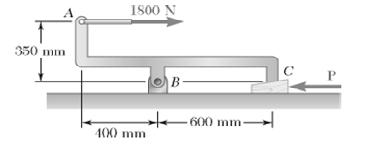# The machine part ABC is supported by a frictionless hinge at B and a 10° wedge at C. Knowing that the coefficient of static friction at both surfaces of the wedge is 0.20, determine (a) the force P required to move the wedge, (b) the components of the corresponding reaction at B.

Question-AnswerCategory: Engineering MechanicsThe machine part ABC is supported by a frictionless hinge at B and a 10° wedge at C. Knowing that the coefficient of static friction at both surfaces of the wedge is 0.20, determine (a) the force P required to move the wedge, (b) the components of the corresponding reaction at B.

The machine part ABC is supported by a frictionless hinge at B and a 10° wedge at C. Knowing that the coefficient of static friction at both surfaces of the wedge is 0.20, determine (a) the force P required to move the wedge, (b) the components of the corresponding reaction at B.